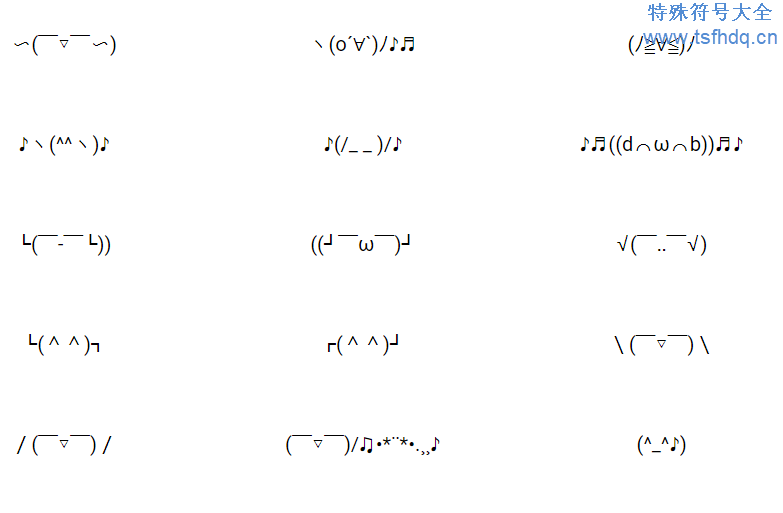# 唱歌跳舞表情符号ヾ(´〇`)ﾉ♪♪♪

ヘ(￣ω￣ヘ)

(〜￣▽￣)〜

〜(￣▽￣〜)

ヽ(o´∀`)ﾉ♪♬

(ﾉ≧∀≦)ﾉ

♪ヽ(^^ヽ)♪

♪(/_ _ )/♪

♪♬((d⌒ω⌒b))♬♪

└(￣-￣└))

((┘￣ω￣)┘

√(￣‥￣√)

└(^^)┐

┌(^^)┘

\(￣▽￣)\

/(￣▽￣)/

(￣▽￣)/♫•*¨*•.¸¸♪

(^_^♪)

(~˘▽˘)~

~(˘▽˘~)

ヾ(⌐■_■)ノ♪

(〜￣△￣)〜

(~‾▽‾)~

~(˘▽˘)~

(｢• ω •)｢

⁽⁽◝( • ω • )◜⁾⁾

✺◟( • ω • )◞✺

♬♫♪◖(● o ●)◗♪♫♬

( ˘ ɜ˘) ♬♪♫

♪♪♪ ヽ(ˇ∀ˇ )ゞ

(ˇ▽ˇ)ノ♪♬♫